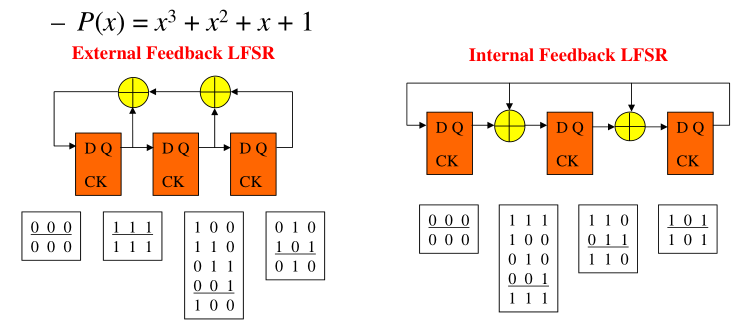# LFSR和特征函数

## 一、LFSR是什么

LFSR有两者类型，外部反馈型和内部反馈型：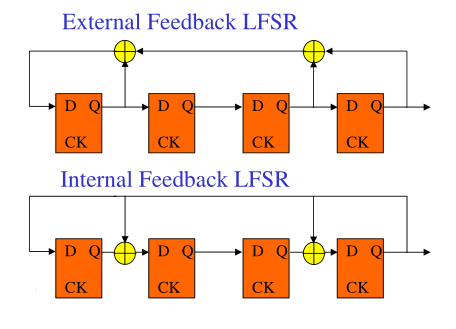$$图 1$$

1 1000 1000
2 1100 0100
3 1110 0010
4 0111 0001
5 0011 1101
6 0001 1110
7 1000 1000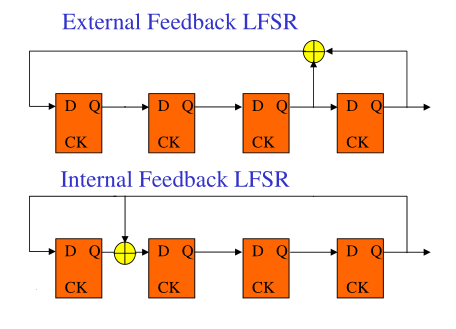$$图 2$$

1 1000 1000
2 0100 0100
3 0010 0010
4 1001 0001
5 1100 1100
6 0110 0110
7 1011 0011
8 0101 1101
9 1010 1010
10 1101 0101
11 1110 1110
12 1111 0111
13 0111 1111
14 0011 1011
15 0001 1001
16 1000 1000

## 二、特征函数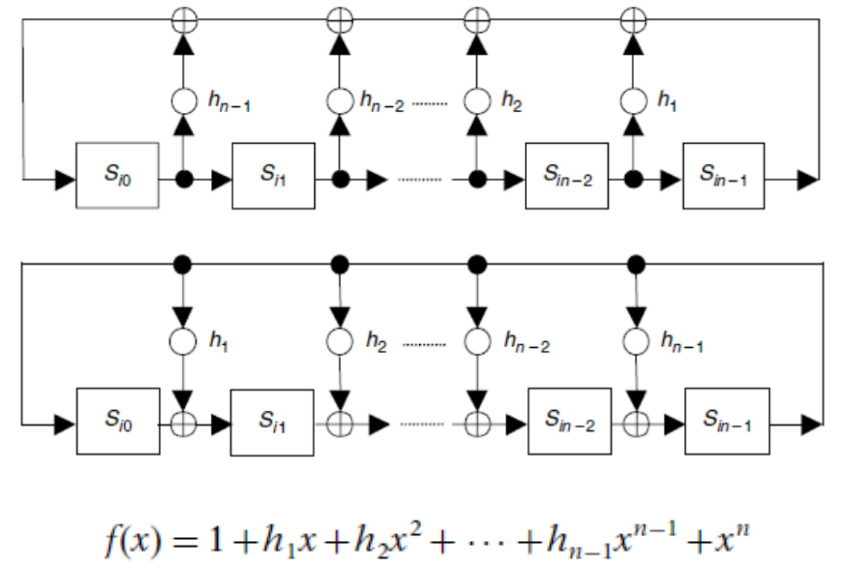\begin{aligned} f_1(x)&=x^4+x^3+x+1\\ f_2(x)&=x^4+x+1 \end{aligned}

### 1、判断周期

$$x^6+1=(x^4+x^3+x+1)(x^2+x+1)$$

$$x^{15}+1=(x^4+x+1)(x^{11}+x^8+x^7+x^5+x^3+x^2+x+1)$$

### 2、计算下一个向量序列

$$S_1=(S_0 *x) \% f(x)$$

$$S_1=(x^2+x^3)x \% (x^4+x+1)=(x^4+x^3)\%(x^4+x+1)=x^3+x+1=1101$$

### 3、由本征f(x)构造伪随机数电路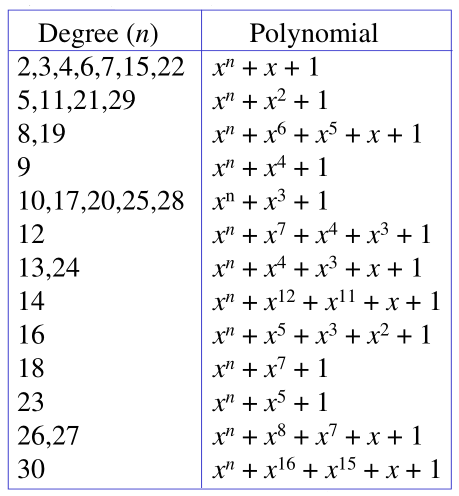## 三、EF和IF关系